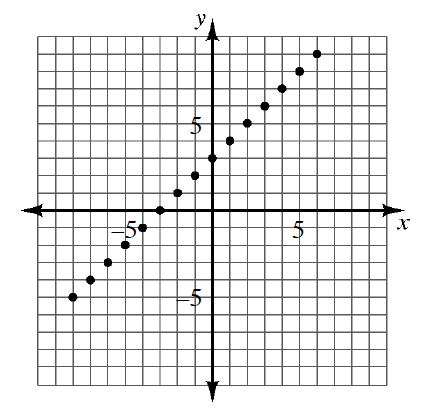### Home > CC3MN > Chapter 3 > Lesson 3.1.5 > Problem3-46

3-46.Create an $x→y$ table using at least eight points from the graph at the right. Write the rule for the pattern in the table.

Rule: __________

Find a pattern in the points from the graph. Then write the rule.

What is the growth rate? Where does this go in the rule?

What is the starting point? Where is this in the rule?

Rule: $y=x+3$